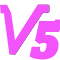磁阻式电磁炮的最优加速度分配

这篇帖子要解决的问题通俗的讲就是，我是把更多电容放在前面效率高，还是放在后面效率高。

首先把问题变成这个形式：对于一个磁阻式电磁炮，已知弹丸初速为v0，出速为v1（v1>v0）。总加速距离为x1（x1>0）。求如何分配各级的加速度，能使效率最高。

引入一个前提条件：整个系统的电阻损耗功率Pr和加速度a的平方成正比，且与其它变量（比如弹丸正在哪一级的哪个位置）无关，即$P_{r}=k\ a^{2}$

这个前提条件对应的实际情况大概是：弹丸始终在磁饱和状态下；分级足够精细，各级紧密相邻，且各级均带关断与能量回收。

结论是：若加速度$a=(C_{3}\ x+C_{4})^{\frac{1}{3}}$，则效率可能最高。其中C3和C4是和v0，v1，x1有关的常数。

求解过程如下：

以位移为零的时刻为时间起点，即$t|_{x=0}=0$，设位移为x1时，时间为t1（t1不是已知量）。设电阻损耗的总能量为$E_{r}$。则此问题可以描述为如下形式：

在满足固定边界条件$v|_{x=0}=v_{0},\ v|_{x=x_{1}}=v_{1}$的情况下，求函数v(x)，使泛函$E_{r}[v(x)]=k\ \int_{0}^{t_{1}} a^{2}\mathrm{d}t$取极小值。

∵$v={\mathrm{d}x}/{\mathrm{d}t},\ a={\mathrm{d}v}/{\mathrm{d}t}$

∴$\mathrm{d}t={\mathrm{d}x}/{v},\ a=v\cdot {\mathrm{d}v}/{\mathrm{d}x}=v\cdot v^{\prime},\ (v^{\prime}={\mathrm{d}v}/{\mathrm{d}x})$

∴$E_{r}[v(x)]=k\int_{0}^{x_{1}}v{\cdot} v^{\prime2}\mathrm{d}x$

设$G(x,v,v^{\prime})=v\cdot v^{\prime2}$

对于物理可实现的系统，$G(x,v,v^{\prime})$拥有连续的二阶偏导数

∴由欧拉—拉格朗日方程可知，Er取极值的必要条件是

$$G_{v}-\frac{\mathrm{d}}{\mathrm{d}x}G_{v^{\prime}}=0$$

$$v^{\prime2}+2v{\cdot} v^{\prime\prime}=0$$

这是一个可降阶的二阶微分方程，可以求出

$$v^{\prime}=C_{0}v^{-\frac{1}{2}}$$

进而求出

$$v=(C_{1}x+C_{2})^{\frac{2}{3}}$$

代入边界条件可以求出C1，C2的值，求出的结果形式上比较复杂 ，mathematica给出的结果是这样的，里面可能有些解不符实际需要舍去

求出v(x)后，可以很方便的求出加速度与位置的关系，即a(x)

$$a(x)=\frac{2}{3}C_{1}(C_{1}x+C_{2})^{\frac{1}{3}}=(C_{3}\ x+C_{4})^{\frac{1}{3}}$$

对于常见的初始速度为0的情况，易知C2=0，所以对于这种情况有

$$a=C_{5}\ x^{\frac{1}{3}}$$

所以初速为0时，若加速度与位置的三分之一次方成正比，则可能得到最高的效率。

至此求解完毕

然后说点闲话。记得我之前曾经发过一篇帖子说效率最高的是匀加速，当时没有发解法（当时本来是打算把另一个问题解决之后一起发出来的，后来发现另一个问题解不动……）结果前两天突然发现，我把当时的解法给忘了……然后又翻了一遍书重做了一遍，发现当时的结论不对，估计是边界条件给错了。当然这个也不保证对，毕竟欧拉方程只给出必要条件，是不是充分条件还要另求……而且关于位移，速度，加速度的那个部分我还有点迷糊……

[修改于 2 年前 - 2018-06-23 13:13:36]

来自：高压与强磁 / 电磁炮

5
2018-6-26 17:49:569524212141楼

看不懂(´･_･`)，等大佬铺好路摸着大佬过河。

1
加载评论中，请稍候...
2020-6-17 23:57:4710班陈大葱22号2楼

楼主牛逼，数学基础真好

这么看来，在第一级就不需要放太多努力了，用细线把电感往高了放，后续的加速级则加粗线径把电感降低，是最合适的

加载评论中，请稍候...
2020-06-23 23:50:32
2020-6-23 23:50:32月下孤狼3楼

磁阻式在理论上比较美好，但是最大的问题是涡电流。目前所用的发射物都是高电导率的铁之类的，涡电流不能忽略，尤其是后面几级，通电时间很短，此时涡电流引起的排斥力会有负面效应。

基于“速度快了通电时间非常短（几十us~ms）”原则上，爱好者的条件下能实现高速的只有多级感应式。从目前能够获取的一些资料来看，实现超高速的线圈炮均为感应式(国外），因为只有感应式是直面“涡电流效应”。

加载评论中，请稍候...
2020-06-24 12:04:35三水合番（作者）
4楼

涡流不是啥大问题

磁阻炮即使有涡流，影响也不是特别显著。因为弹丸口径较小，电阻率也比铜铝这种高很多。所以弹丸时间常数很小，一般只有十几us级，就算速度到200m/s，也依然显著小于线圈电流脉冲的持续时间，所以涡流不会造成倍数级别的性能恶化。“你好，电磁炮”那本书上有考虑涡流和不考虑涡流的仿真对比，速度差距并不显著。

而且磁阻炮在原理上也不是必然产生涡流的。它产生电磁力，只需要弹丸两端有磁通量差，不需要弹丸上的磁场有波动。所以只要分级足够细密，就可以通过“减小磁场波动”的方式，来减小涡流。参考这篇帖子 https://www.kechuang.org/t/81534 在使用这种方案实现高速高效率的过程中，涡流的问题自然而然的就解决了，不是啥大问题

爱好者只有多级感应炮实现了高速，也不是因为它直面了涡流。只是因为感应炮的效率和加速度无关，可以靠堆储能的方式，大力出奇迹，用较少的级数达到高速，而不会让像磁阻炮那样让效率降到ppm级。

另外，涡流的问题和本文主题无关，有兴趣的话建议到这篇帖子下讨论 https://www.kechuang.org/t/81534

加载评论中，请稍候...

想参与大家的讨论？现在就 登录 或者 注册三水合番进士 专家 学者 机友 笔友
文章
39
回复
773
学术分
2
2014/04/30注册，2 小时前活动

从事基于天然神经网络的天线拓扑优化

相似文章推荐
插入资源
全部
图片
视频
音频
附件
全部
未使用
已使用
正在上传
空空如也~
上传中..{{f.progress}}%
处理中..
上传失败，点击重试
{{f.name}}
空空如也~
(视频){{r.oname}}
{{selectedResourcesId.indexOf(r.rid) + 1}}
插入表情
我的表情
共享表情
Emoji
上传
##### 注意事项
最大尺寸100px，超过会被压缩。为保证效果，建议上传前自行处理。
建议上传自己DIY的表情，严禁上传侵权内容。
点击重试等待上传{{s.progress}}%处理中...已上传
空空如也~
草稿箱
加载中...
此处只插入正文，如果要使用草稿中的其余内容，请点击继续创作。
{{fromNow(d.toc)}}
{{getDraftInfo(d)}}
标题：{{d.t}}
内容：{{d.c}}
{{forum.displayName}}